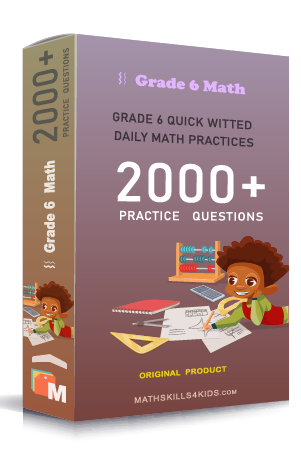# Grade 6 Two-variable equations worksheets with solutions

Subject
Math

Resource Type
Worksheets, Printables, Homeschool

Standards
CCSS 6.EE.B.5 | CCSS 6.EE.B.6 | CCSS 6.EE.C.9

Format
PDF (20.2 MB | 28 pages → Exercises + Solutions)

• ### -15% OFF Over \$100

Promo Code: TOPLA

After Purchase

\$3.45

This package allows you to practice the following skills

Does (x,y) satisfy an equation?

Interpret a graph word problems

Writing an equation from a graph using a table

Identifying independent and dependant variables

Finding a value using two-variable equations

Using two-variable equations to find a value word problems

Completing a table for a two-variable relationship

Writing two-variable equations

Identifying the graph of two-variable equations

Graph a two-variable equation

This product is a part of the Mega Pack
* Grade 6 quick-witted daily math practices *